# Frenkel line

(diff) ← Older revision | Latest revision (diff) | Newer revision → (diff)

The Frenkel line is a line of change of thermodynamics, dynamics and structure of fluids. Below the Frenkel line the fluids are "rigid" and "solid-like" while above it fluids are "soft" and "gas-like".

Two types of approaches to the behavior of liquids are present in the literature. The most common one is due to van der Waals. It treats the liquids as dense structureless gases. Although this approach allows one to explain many principle features of fluids, in particular, the liquid-gas phase transition, it fails to explain other important issues such as, for example, the existence in liquids of transverse collective excitations such as phonons.

Another approach to fluid properties was proposed by Jacov Frenkel . It is based on the assumption that at moderate temperatures the particles of liquid behave in a similar manner as a crystal, i.e. the particles demonstrate oscillatory motions. However, while in crystal they oscillate around theirs nodes, in liquids after several periods the particles change the nodes. This approach is based on postulation of some similarity between crystals and liquids, providing insight into many important properties of the latter: transverse collective excitations, large heat capacity and so on.

From the discussion above one can see that the microscopic behavior of particles of moderate and high temperature fluids is qualitatively different. If one heats up a fluid from a temperature close to the melting point up to some high temperature, a crossover from the solid-like to gas-like regime appears. The line of this crossover was named Frenkel line after J. Frenkel.

Several methods to locate the Frenkel line were proposed in the literature. The most detailed reviews of the methods are given in Refs. , . The exact criterion of Frenkel line is the one based on comparison of characteristic times in fluids. One can define a 'jump time' via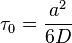$\tau_0=\frac{a^2}{6D}$,

where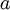$a$ is the particles size and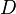$D$ is the diffusion coefficient. This is the time necessary for a particle to move a distance comparable to it's own size. The second characteristic time corresponds to the shortest period of transverse oscillations of particles within the fluid,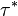$\tau^*$. When these two time scales are roughly equal one cannot distinguish between the oscillations of the particles and theirs jumps to another position. Thus the criterion for Frenkel line is given by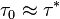$\tau_0 \approx \tau^*$.

There are several approximate criteria to locate the Frenkel line on the pressure-temperature plane (see Refs. , , ). One of these criteria is based on the velocity autocorrelation function (vacf): below the Frenkel line the vacf demonstrates oscillatory behaviour, while above it the vacf monotonically decays to zero. The second criteria is based on the fact that at moderate temperatures liquids can sustain transverse excitations, which disappear upon heating. One further criteria is based on isochoric heat capacity measurements. The isochoric heat capacity per particle of a monatomic liquid near to the melting line is close to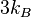$3 k_B$ (where$k_B$ is the Boltzmann constant). The contribution to the heat capacity due to potential part of transverse excitations is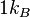$1 k_B$. Therefore at the Frenkel line, where transverse excitations vanish, the isochoric heat capacity per particle should be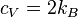$c_V=2 k_B$, a direct prediction from the phonon theory of liquid thermodynamics   .

Crossing the Frenkel line leads also to some structural crossovers in fluids  . Currently Frenkel lines of several idealised liquids, such as Lennard-Jones and soft spheres , ,  as well as realistic models such as liquid iron , hydrogen , water , carbon dioxide , and methane  have been reported in the literature.